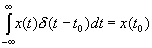# More Examples of Continuous-Time Convolution

Now, what if we convolve x(t) with an impulse?

Example 2 Find x(t) *  δ(t - t0)  =  y(t),

Now, δ(t - τ - t0) is an impulse at τ = t - t0. So, by the SIFTING property, we sift out the value of x(t) where the impulse occurs. This is just x(t - t0).

So, if you convolve x(t) with a shifted impulse, you just get the function x(t) shifted to where the impulse occurs.

In other words,

x(t) * δ(t -  t0) = x(t - t0)

We'll see this a lot. One important place we'll see this is when we discuss SAMPLING or discretizing a continuous time signal.

NOW, IN EXAMPLE 3, YOU WILL BE ASKED TO WORK THROUGH THE PROBLEM STEP-BY-STEP.

FOR ADDITIONAL PRACTICE IN PERFORMING CONVOLUTION, WORK EACH EXAMPLE TWICE. THE FIRST TIME, FLIP-AND-SHIFT x(t), AND THE SECOND TIME FLIP-AND-SHIFT h(t).

Example 3 Since convolution is commutative, do this example twice. One time pick the unit step function to time reverse and the next time, pick the exponential.

From before:

Sifting property of impulse:"This sifts out the value of the function x(t) where impulse occurs"

Example 4

Example 5

Example 6